# The box

The box contains three light bulbs with a wattage of 40 W and two pieces of light bulbs with a wattage of 60 W. What is the probability of the event that two randomly selected light bulbs will both be 40 W?

p =  0.3

### Step-by-step explanation:Did you find an error or inaccuracy? Feel free to write us. Thank you!Tips to related online calculators
Would you like to compute count of combinations?

## Related math problems and questions:

• Bulbs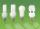In the box are 6 bulbs with power 75 W, 14 bulbs with power 40 W and 15 with 60 W. Calculate probability that a randomly selected bulb is:
• Screws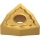The box contains 8 iron, 6 brass, and 4 titanium screws. What is the probability that the randomly selected screw will not be brass?
• Electric energyThere are 875 identical light bulbs in the sports hall lighting for 2 hours. How long does the same amount of electricity consume 100 such bulbs?
• CardsFrom a set of 32 cards, we randomly pull out three cards. What is the probability that it will be seven kings and ace?
• Two trains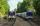Two trains go at each other. First at speed 40 km/h and the second at speed 60 km/h. At what distance will be 30 minutes before they will meet?
• Triangle ABCConstruct a triangle ABC is is given c = 60mm hc = 40 mm and b = 48 mm analysis procedure steps construction
• LifespanThe lifetime of a light bulb is a random variable with a normal distribution of x = 300 hours, σ = 35 hours. a) What is the probability that a randomly selected light bulb will have a lifespan of more than 320 hours? b) Up to what value of L hours can the
• Events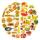Event P has probability of 0.84. What is the probability that the event P occurs in 3, 5, 7 try.
• Raffle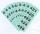In the pool is numbers from 1 to 115. What is the probability that a randomly selected number is not a prime number?
• White and black ballsThere are 7 white and 3 black balls in an opaque pocket. The balls are the same size. a) Randomly pull out one ball. What is the probability that it will be white? We pull out one ball, see what color it is and return it to the pocket. Then we pull out th
• Two diceWe roll two dice. What is the probability that the sum of the falling numbers is greater than 3?
• The bulbsThe bulbs are connected serially two bulbs and one resistor. Each bulb has a resistivity of 100 ohms and resistor of 60 ohms The voltage source in the circuit is 24 volts. Do you know what voltage to measure on individual appliances?
• Class - boys and girls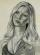In the class are 60% boys and 40% girls. Long hair has 10% boys and 80% girls. a) What is the probability that a randomly chosen person has long hair? b) The selected person has long hair. What is the probability that it is a girl?
• Bulbs and electricity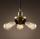In the sports hall, 875 identical light bulbs light for 2 hours. How long does it take for 100 such light bulbs to consume the same amount of electricity?
• The box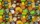The box contains five chocolate, three fruit, and two menthol candies. We choose sweets at random from the box. What probability will we take out one chocolate, one fruit, and one menthol candy without a return?
• Event probability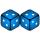The probability of event P in 8 independent experiments is 0.33. What is the probability that the event P occurs in one experiment (chance is the same)?
• Bulb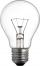A 60 W bulb consumes 0.12 kWh of electricity. How long did she stand light without a break?